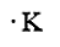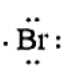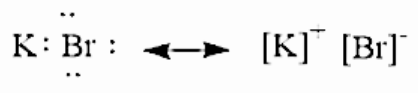# How would you represent potassium and bromine using the diagram? How about KBr?Verified
179.4k+ views
Hint:In order to represent an atom or molecule in an electron dot structure, we must know about things, i.e., one is the chemical symbol of the element and the other is the number of valence electrons present in it.

Let us understand what an electron dot structure is. Electron dot structure referred to as Lewis structure. Electron dot structure is the diagram which will tell about the bonding between the atoms present in a molecule. In the electron dot structure, each dot will represent the electron. For giving an electron dot structure, we must be knowing about two things, one is the atom’s chemical symbol and the other one is the number of valence electrons present in it.
Let us first start with a chemical element, i.e., Potassium which is present in Group 1 and period 4 of the periodic table. The electronic configuration of Potassium is given as
$K = 1{s^2}2{s^2}2{p^6}3{s^2}3{p^6}4{s^1}$
The number of valence electrons present in Potassium is 1. The electron dot structure of Potassium is given asNow let us consider a chemical element, i.e., Bromine which is present in Group 17 and period 4 of the periodic table. The electronic configuration of Bromine is given as $1{s^2}2{s^2}2{p^6}3{s^2}3{p^6}4{s^2}4{p^5}$. The number of valence electrons present in Bromine is 7. The electron dot structure of Bromine is given asWhen the potassium and Bromine combine together, the more electronegative Bromine will pull the valence electron of the Potassium atom towards itself.Note:
We have to remember that potassium bromide has various uses. It can be used as
- Sedative
- Anticonvulsant
- Manufacture of chemicals
- plasticizers
- laboratory agents